Lakhmir Singh & Manjit Kaur: Gravitation, Solutions- 3

# Lakhmir Singh & Manjit Kaur: Gravitation, Solutions- 3 - Science Class 9

Page No - 123

Question 1:
Write the common unit of density.
Solution :
Grams per cubic centimtre (g/cm3).

Question 2:
What is the density of water in SI units ?
Solution :
Density of water =1000kg/m3.

Question 3:
What is the value of relative density of water ?
Solution :
Relative density of water is 1.

Question 4:
Name the quantity whose one of the units is pascal (Pa).
Solution :
Pressure has unit of Pascal (Pa).

Question 5:
State the units in which pressure is measured.
Solution :
Pressure is measured in newtons per square metre (N/m2) i.e., pascal (Pa).

Question 6:
State whether the following statements are true or false :
(a) The buoyant force depends on the nature of object immersed in the liquid
(b) Archimedes’ principle can also be applied to gases.

Solution :
(a) False
(b) True

Question 7:
In which direction does the buoyant force on an object due to a liquid act ?
Solution :
Buoyant force on an object due to a liquid act s in the vertically upward direction.

Question 8:
What is the other name of buoyant force ?
Solution :
Upthrust is the other name of buoyant force.

Question 9:
Name the force which makes heavy objects appear light when immersed in a liquid.
Solution :
Buoyant force.

Question 10:
What is upthrust ?
Solution :
The upward force acting on an object immersed in a liquid is called upthrust.

Question 11:
Name the principle which gives the magnitude of buoyant force acting on an object immersed in a liquid.
Solution :
Archimedes’ Principle.

Question 12:
lire relative density of mercury is 13.6. What does this statement mean ?
Solution :
The relative density of mercury is 13.6, this means that mercury is 13.6 times as heavy as an equal volume of water.

Question 13:
What name is given to ‘thrust per unit area’ ?
Solution :
Pressure is ‘thrust per unit area’.

Question 14:
What is the ss ientific name of the ‘upward force’ acting on an object immersed in a liquid ?
Solution :
Buoyant force or upthrust.

Question 15:
What is meant by the term ‘buoyancy’ ?
Solution :
The tendency of a liquid to exert an upward force on an object placed in it, is called buoyancy.

Question 16:
What causes buoyant force (or upthrust) on a boat ?
Solution :
The buoyant force on a boat is caused by the pressure of water ‘pushing up’ on the bottom of the boat.

Question 17:
Why does ice float in water ?
Solution :
The density of ice is less than that of water, so ice floats in water.

Question 18:
What force acting on an area of 0.5 m2 will produce a pressure of 500 Pa
Solution :
Force = Pressure x Area
Force = 500 x 0.5 = 250 N

Question 19:
An object of weight 200 N is floating in a liquid. What is the magnitude of buoyant force acting on it ?
Solution :
Since the object floats in the liquid, so the magnitude of the buoyant force exerted by the liquid is equal to the weight of the object.
Hence, buoyant force =200N

Question 20:
Name the scientist who gave he magnitude of buoyant force acting on a solid object immersed in a liquid.
Solution :
Archimedes gave the magnitude of buoyant force acting on a solid object immersed in a liquid

Question 21:
The density of gold is 19 g/cm3. Find the volume of 95 g of gold.
Solution :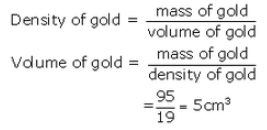Question 22:
What is the mass of 5 m3 of cement of density 3000 kg/m3 ?
Solution :
Volume=5m3
Density= 3000kg/m3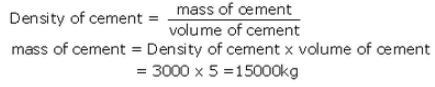Question 23:
What is the density of a substance of mass 100 g and volume 10 cm3 ?
Solution :
Mass of the substance = 100g
Volume of the substance =10cm3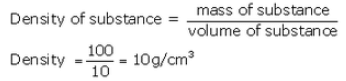Question 24:
Why does a block of wood held under water rise to the surface when released ?
Solution :
Because the weight of the block of wood is less than the weight of an equal volume of water. So when it is completely submerged in water, the upward buoyant force on it is greater than the downward gravitational force on it. Hence, the lock rises to the surface.

Question 25:
The density of a body is 800 kg/m3. Will it sink or float when dipped in a bucket of water ? (Density’ of water = 1000 kg/m3).
Solution :
The body will float when dipped in a bucket of water as its density is less than that of water.

Question 26:
Fill in the following blanks with suitable words :
(a) Force acting on a unit area is called…………………..
(b) It is the……………. force which makes objects appear lighter in water.
(c) A heavy ship floats in water because its……………………. density is less than that of water.
(d) In fluids (liquids and gases), pressure acts in……………………….. directions, and pressure………………… as the depth increases.
(e) In order to sink in a fluid, the density of an object must be……………………… than the………………… of the fluid.
(f) Snow shoes work by spreading out a person’s……………………… over a much bigger………………………..
(g) If the area of a snow shoe is five times……………………. than the area of an ordinary shoe, then the pressure of a snow shoe on the snow is five times……………………

Solution :
(a) pressure
(b) buoyant
(c) average
(d) all; increases
(e) less; density
(f) weight; area
(g) bigger; smaller

Page No - 124

Question 27:
(a) What is the difference between the density and relative density of a substance ?
(b) If the relative density of a substance is 7.1, what will be its density in SI units ?
Solution :
(a) The density of a substance is defined as mass of the substance per unit volume.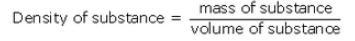SI unit of density is kg/m3

The relative density of a substance is the ration of its density to that of water.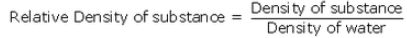It has no unit.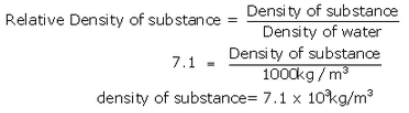Question 28:
Define thrust. What is its unit ?
Solution :
The force acting on a body perpendicular to its surface is called thrust. The SI unit of thrust is newton (N).

Question 29:
A mug full of water appears light as long as it is under water in the bucket than when it is outside water. Why ?
Solution :
A mug full of water appears light as long as it is under water because buoyant force acts on it which reduces its effective weight and makes it appear lighter.

Question 30:
What happens to the buoyant force as more and more volume of a solid object is immersed in a liquid ? When does the buoyant force become maximum ?
Solution :
As more and more volume of the solid object is immersed in the liquid, the upward ‘buoyant force’ also keeps on increasing. When the object is completely immersed in the liquid, the buoyant force acting on the solid becomes maximum and remains constant thereafter.

Question 31:
Why do we feel light on our feet when standing in a swimming pool with water up to our armpits ?
Solution :
As more and more volume of our body is immersed in water, the apparent weight of the body goes on decreasing and the body seems to become lighter. This is due to the increase in upward buoyant force acting on the body.

Question 32:
Explain why, big boulders can be moved easily by flood.
Solution :
Big boulders weig much less while in water and as such are easily moved by the flood.

Question 33:
An iron nail sinks in water but it floats in mercury. Why ?
Solution :
An iron nail sinks in water but it floats in mercury because density of iron is more than that of water but less than that of mercury.

Question 34:
Explain why, a piece of glass sinks in water but it floats in mercury.
Solution :
A piece of glass sinks in water but it floats in mercury because density of glass is more than that of water but less than that of mercury.

Question 35:
Steel sinks in water but a steel boat floats. Why ?
Solution :
A piece of steel sinks in water because steel is denser than water. However, a steel ship is a hollow object made of steel and contains a lot of air in it. Due to presence of a lot of air in it, the average density of the ship becomes less than the density of water. Hence a ship floats in water.

Question 36:
Explain why, school bags are provided with wide straps to carry them.
Solution :
School bags have wide straps so that their weight may spread over a large area of shoulder producing less pressure on the shoulder.

Question 37:
Why does a sharp knife cut objects more effectively than a blunt knife ?
Solution :
A sharp knife cuts objects easily because due to its very thin edge, the force of our hand falls on a very small area of the object producing large pressure.

Question 38:
Explain why, wooden (or concrete) sleepers are kept below the railway line.
Solution :
Concrete or wooden
sleepers are kept below the railway line so that the weight of passing train
is spread over a large area of ground and the track may not sink into the
ground.

Question 39:
Explain why, a wide steel belt is provided over the wheels of an army tank.The density of iron or steel is much higher than that of water, so an object made of iron or steel (like this car) sinks in water.
Solution :
A wide steel belt is provided over the wheels of an army tank so that they exert less pressure on the ground and do not sink into it.

Question 40:
Explain why, the tip of a sewing needle is sharp.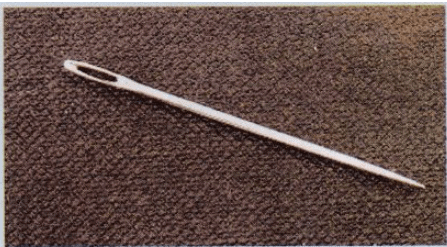Solution :
The tip of the sewing needle is sharp so that due to its sharp tip, the needle may put the force on a very small area of the cloth, producing a large pressure sufficient to pierce the cloth being stitched.

Question 41:
When is the pressure on the ground more—when a man is walking or when a man is standing ? Explain.
Solution :
When a man is walking, then at one time only one foot is on the ground. Due to this, the force of weight of man falls on a smaller area of the ground and produces more pressure on the ground. On the other hand, when the man is standing, then both his feet are on the ground. Due to this, the weight of the man falls on a larger area of the ground and produces lesser pressure on the ground.

Question 42:
Explain why, snow shoes stop you from sinking into soft snow.
Solution :
Snow shoes stop us from sinking into soft snow because due to large area of snow shoes, our weight is spread over a large area of the snow producing small pressure.

Question 43:
Explain why, when a person stands on a cushion, the depression is much more than when he lies down on it.
Solution :
When a person stands on a cushion then only his two feet (having small area) are in contact with the cushion. Due to this the weight of man falls on a small area of the cushion producing a large pressure causing a big depression in the cushion. On the other hand, when the same person lies down on the cushion, then his whole body (having large area) is in contact with the cushion. Here, his weight falls on a much larger area of the cushion producing much smaller pressure and very little depression in the cushion.

Question 44:
Use your ideas about pressure to explain why it is easier to walk on soft sand if you have flat shoes rather than shoes with sharp heels.
Solution :
Flat shoes have greater area in contact with the soft sand as compared to heels. Due to this, there is less pressure on soft sand because of which they do not sink much in the sand and it is easy to walk on it.

Question 45:
Explain why, a nail has a pointed tip.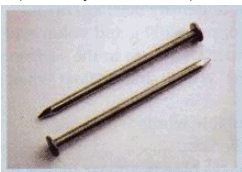A nail has a pointed tip

Solution :
A nail has a pointed tip, so that when it is hammered, the force of hammer is transferred to a very small area of wood creating a large pressure which pushes the nail into the wood.

The document Lakhmir Singh & Manjit Kaur: Gravitation, Solutions- 3 | Science Class 9 is a part of the Class 9 Course Science Class 9.
All you need of Class 9 at this link: Class 9

## Science Class 9

66 videos|355 docs|97 tests

## FAQs on Lakhmir Singh & Manjit Kaur: Gravitation, Solutions- 3 - Science Class 9

 1. What is the formula for the gravitational force between two objects?Ans. The formula for the gravitational force between two objects is given by Newton's law of universal gravitation, which states that the force (F) between two objects is directly proportional to the product of their masses (m1 and m2), and inversely proportional to the square of the distance (r) between their centers. Mathematically, it can be represented as F = G * (m1 * m2) / r^2, where G is the gravitational constant.
 2. How does the gravitational force affect the motion of objects?Ans. The gravitational force affects the motion of objects by causing them to accelerate towards each other. According to Newton's second law of motion, the acceleration of an object is directly proportional to the net force acting on it and inversely proportional to its mass. Since the gravitational force is constant and depends only on the masses of the objects, the acceleration caused by gravity is the same for all objects near the Earth's surface, resulting in the familiar phenomenon of objects falling towards the ground.
 3. What is the difference between mass and weight?Ans. Mass and weight are two different physical quantities. Mass is a measure of the amount of matter present in an object and is constant, regardless of the location. It is usually measured in kilograms. On the other hand, weight is the force exerted on an object due to gravity. It depends on the mass of the object and the strength of the gravitational field. Weight is usually measured in newtons. For example, an object with a mass of 10 kg will have a weight of approximately 98 newtons on Earth's surface.
 4. How does the gravitational force change with distance?Ans. The gravitational force between two objects decreases as the distance between them increases. According to Newton's law of universal gravitation, the force is inversely proportional to the square of the distance between their centers. This means that if the distance between two objects is doubled, the gravitational force between them will decrease by a factor of four. Similarly, if the distance is tripled, the force will decrease by a factor of nine. Therefore, the gravitational force weakens as the distance increases.
 5. What is the significance of the gravitational constant (G)?Ans. The gravitational constant (G) is a fundamental constant in physics that determines the strength of the gravitational force between two objects. Its value is approximately 6.674 × 10^-11 N m^2/kg^2. The significance of the gravitational constant lies in its role in quantifying the strength of gravity and allowing us to calculate the gravitational force accurately. Without the gravitational constant, we would not be able to describe or understand the behavior of objects under the influence of gravity.

## Science Class 9

66 videos|355 docs|97 testsExplore Courses for Class 9 examSignup to see your scores go up within 7 days! Learn & Practice with 1000+ FREE Notes, Videos & Tests.
10M+ students study on EduRev
Track your progress, build streaks, highlight & save important lessons and more!
Related Searches

,

,

,

,

,

,

,

,

,

,

,

,

,

,

,

,

,

,

,

,

,

,

,

,

;